# Texas Go Math Grade 3 Lesson 16.3 Answer Key Area of Rectangles

Refer to our Texas Go Math Grade 3 Answer Key Pdf to score good marks in the exams. Test yourself by practicing the problems from Texas Go Math Grade 3 Lesson 16.3 Answer Key Area of Rectangles.

## Texas Go Math Grade 3 Lesson 16.3 Answer Key Area of Rectangles

Unlock the Problem

The rectangles show Mr. Koi’s plans for storage sheds. How are the areas of the sheds related? How will the areas of Sheds A and B change? How will the areas of Sheds C and D change?What do I need to find?

I need to find how the areas will change fromA to Band from _________ to _________.

What information am I given?

I am given the _________ and _________ of each shed to find its area.

Plan

What is my plan or strategy?

I will record the areas in a table. Then I will look for a pattern to see how the __________ will change.

Solve

I will complete the table to find patterns to solve the problem.The areas change from _________ to _________ and from _________ to _________. see that the lengths will be the same and the widths will be doubled. So, when the lengths are the same and the widths are doubled, the areas will be _________.

What do I need to find?

I need to find how the areas will change from A to B and from C to D.

What information am I given?

I am given the Length  and Width of each shed to find its area.

Plan

What is my plan or strategy?

I will record the areas in a table. Then I will look for a pattern to see how the Area will change.

Solve

I will complete the table to find patterns to solve the problem.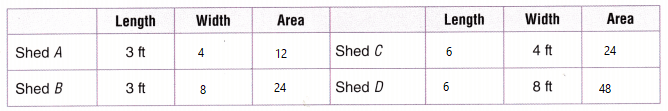The areas change from 12 to 24 and from 24 to 48. see that the lengths will be the same and the widths will be doubled. So, when the lengths are the same and the widths are doubled, the areas will be Double.

Try Another Problem

Mr. Koi is planning more storage sheds. He wants to know how the areas of the sheds are related. How will the areas of Sheds E and F change? How will the areas of Sheds G and H change?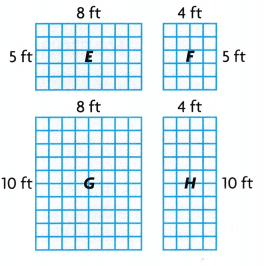What do I need to find?

What information am I given?

Plan

What is my plan or strategy?

Solve

I will complete the table to find patterns to solve the problem.What do I need to find?
I need to find how the areas will change from E to F and from G to H.

What information am I given?
I am given the Length  and Width of each shed to find its area.

Plan

What is my plan or strategy?
I will record the areas in a table. Then I will look for a pattern to see how the Area will change.

Solve

I will complete the table to find patterns to solve the problem.The areas change from 40 to 20 and from 80 to 40. see that the lengths will be the same and the widths will be Halved . So, when the lengths are the same and the widths are halved, the areas will be halves.

What if both the lengths anti widths of the sheds are doubled? How would the areas change?
Answer: The area will increase 4 times

Share and Show

Use the table for 1-2.Question 1.
Many swimming Pools are built in rectangular shapes. How do the areas of the pools in the table change when the lengths change?
The __________ stays the same.
The lengths ____________.
The areas ________ by __________ square feet.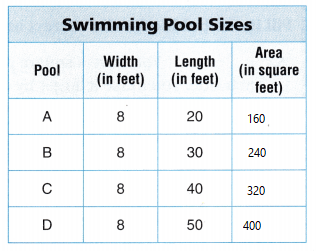Explanation:
The Width stays the same.
The lengths Change
The areas Change by 80 square feet.

What if the width of each pool was 4 feet? Explain how the areas would change

Explanation:
If the width stays the same as 4
The length change
The area changed by 40

Problem Solving

Question 3.
H.O.T. Apply Elizabeth built a sandbox that is 4 feet long and 4 feet wide. She also built a flower garden that is 4 feet long and 6 feet wide. She built a vegetable garden that is 4 feet long and 8 feet wide. Explain how the areas change.Explanation:
The length is same
The width change
Area of sandbox is 16
Area of Flower garden is 24
Area of vegetable garden is 32
Area will change by 8

Question 4.
H.O.T. Reasoning Jacob has a rectangular garden with an area of 56 square feet. The length of the garden is 8 feet. What is the width of the garden? Hint: Use an array of unit squares.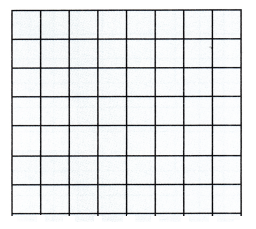Explanation:
The width of the garden is 7
The array of unit squares shows the Area is 54
and the length is 8

Fill in the bubble for the correct answer choice.

Question 5.
Multi-Step You have a square photo that is 2 inches on each side. You make it larger by doubling each side. When you double each side again. How do the areas change?
(A) They stay the same.
(B) They are 4 times greater.
(C) They double.
(D) They are 3 times greater.
Explanation:
The area change by 4 times greater
2 x 2 = 4
4 x 4 = 16
8 x 8 = 64

Question 6.
Lou makes rugs for an art fair. Each unit square is 1 square foot. Lou continues the pattern shown. What is the area in square feet of his next rug?(A) 25 square feet
(B) 8 square feet
(C) 30 square feet
(D) 32 square feet
Explanation:
First rug area is 2 x 2 = 4
second rug area is 3 x 3 = 9
third rug area is 4 x 4 = 16
fourth rug is 5 x 5 = 25 square feet

A rectangle is 40 centimeters long and 4 centimeters wide. Double one number and halve the other. What will be the area of the rectangle that you get after repeating the process 3 times?
(A) 120 square centimeters
(B) 240 square centimeters
(C) 320 square centimeters
(D) 160 square centimeters
Explanation:
Doubled the one number and halve the other Number
40 is halved to 20 and it is again halved to 10 and it is again halved to 5
4 is doubled to 8 and again it is doubled to 16 and again it is doubled to 32
32 x 5 = 160 Square Centimeters.

Texas Test Prep

Question 8.
Caroline mows the three lawns shown in the table. How do the areas change from one row to the next?(A) The areas double.
(B) The areas do not change.
(C) The areas increase by 4 square yards.
(D) The area increased by 32 square yards.
Explanation:
4 x 8 = 32
8 x 8 = 64
12 x 8 = 96 The areas increase by 32 square yards.

### Texas Go Math Grade 3 Lesson 16.3 Homework and Practice Answer Key

Use the table for 1-2.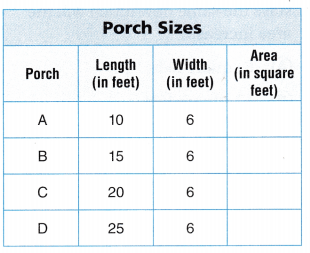Question 1.
A company builds wooden porches. The porches are built in rectangular shapes. How do the areas of the porches change when the lengths change?
The _________ stays the same.
The lengths ___________.
The areas _________ by ________ square feet.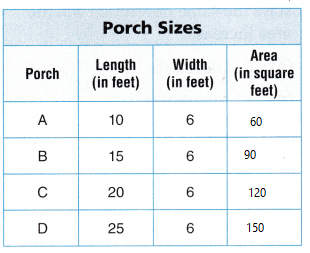Explanation:
The Width stays the same.
The lengths Change
The areas Change by 30 square feet.

Question 2.
What if 2 feet were added to each width? Explain how the area of each porch would change.Explanation:
The Width stays the same.
The lengths Change
The areas Change by 40 square feet.

Problem Solving

Question 3.
An artist paints a mural that is 3 feet long and 5 feet wide. She paints a second mural that is 5 feet long and 5 feet wide. A third mural is 7 feet long and 5 feet wide. Explain how the areas of the murals change.
Explanation:
3 x 5 = 15
5 x 5 = 25
7 x 5 = 35
The areas of the murals change by 10

Question 4.
A flag has a total area of 42 square feet. A second flag has an area of 48 square feet. 1f the widths of both flags are each 6 feet, what are the lengths? Explain.
Answer: The lengths are 7 and 8
Explanation:
The width is same
the length change
6 x 8 = 48
6 x 7 = 42

Lesson Check

Question 5.
Multi-Step Hideki makes a quilt that has a length of 6 feet and an area of 36 feet. If he increases the length to 8 feet, and the width stays the same how much will the area increase?
(A) 6 square feet
(B) 12 square feet
(C) 48 square feet
(D) 20 square feet
Explanation:
The area increased by 12 square feet
6 x 6 = 36
6 x 8 = 48
48 – 36 = 12

Marina makes tablecloths. Each unit square is I square meter. She continues the pattern shown below.What is the area of the next tablecloth?
(A) 9 square meters
(B) 15 square meters
(C) 12 square meters
(D) 18 square meters
Explanation:
3 x 1 = 3
3 x 2 = 6
3 x 3 = 9
3 x 4 = 12
The area of next tablecloth is 12 square meters

Question 7.
A company makes tarps in the sizes shown in the table. How do the areas change?(A) They do not change.
(B) They increase by 4 square meters.
(C) They increase by 6 square meters.
(D) They increase by 8 square meters.
Explanation:
3 x 6 = 18
4 x 6 = 24
4 x 8 = 32
The areas increase by 6 square meters.

Question 8.
Multi-Step Maya has a paint canvas that is 2 feet long and 4 feet wide. Another canvas is double the length and width of the first canvas. How does the area change?
(A) It is doubled.
(B) It is 3 times greater.
(C) It is tripled.
(D) It is 4 times greater.
Explanation:
2 x 4 = 8
4 x 8 = 32
The area will be Doubled

Question 9.
Multi-Step A rectangle is 20 centimeters long and 6 centimeters wide. Double the width and halve the length. What is the area of the new rectangle?
(A) 70 square centimeters
(B) 120 square centimeters
(C) 90 square centimeters
(D) 100 square centimeters